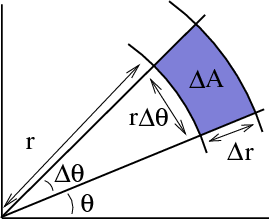# Math Insight

### Image: Area calculation for changing variables to polar coordinatesThe area $\Delta A$ of a blue region in the $xy$-plane corresponding to a $\Delta r \times \Delta\theta$ rectangle in the $r\theta$-plane is approximately $\Delta A \approx r \Delta r \Delta\theta$.

Image file: polar_coordinates_area_calculation.png